# Search by Topic

#### Resources tagged with Ratio similar to Rarity:

Filter by: Content type:
Age range:
Challenge level:

### There are 36 results

Broad Topics > Fractions, Decimals, Percentages, Ratio and Proportion > Ratio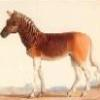### Rarity

##### Age 16 to 18 Challenge Level:

Show that it is rare for a ratio of ratios to be rational.### Mixing More Paints

##### Age 14 to 16 Challenge Level:

Can you find an efficent way to mix paints in any ratio?### Pythagoras’ Comma

##### Age 14 to 16 Challenge Level:

Using an understanding that 1:2 and 2:3 were good ratios, start with a length and keep reducing it to 2/3 of itself. Each time that took the length under 1/2 they doubled it to get back within range.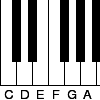### Tuning and Ratio

##### Age 16 to 18 Challenge Level:

Why is the modern piano tuned using an equal tempered scale and what has this got to do with logarithms?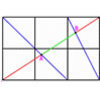### All about Ratios

##### Age 16 to 18 Challenge Level:

A new problem posed by Lyndon Baker who has devised many NRICH problems over the years.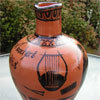### Six Notes All Nice Ratios

##### Age 14 to 16 Challenge Level:

The Pythagoreans noticed that nice simple ratios of string length made nice sounds together.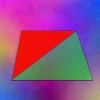### Same Height

##### Age 14 to 16 Challenge Level:

A trapezium is divided into four triangles by its diagonals. Can you work out the area of the trapezium?### Pent

##### Age 14 to 18 Challenge Level:

The diagram shows a regular pentagon with sides of unit length. Find all the angles in the diagram. Prove that the quadrilateral shown in red is a rhombus.### Equal Temperament

##### Age 14 to 16 Challenge Level:

The scale on a piano does something clever : the ratio (interval) between any adjacent points on the scale is equal. If you play any note, twelve points higher will be exactly an octave on.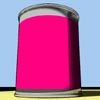### Tin Tight

##### Age 14 to 16 Challenge Level:

What's the most efficient proportion for a 1 litre tin of paint?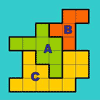### The Rescaled Map

##### Age 14 to 16 Challenge Level:

We use statistics to give ourselves an informed view on a subject of interest. This problem explores how to scale countries on a map to represent characteristics other than land area.### One and Three

##### Age 14 to 16 Challenge Level:

Two motorboats travelling up and down a lake at constant speeds leave opposite ends A and B at the same instant, passing each other, for the first time 600 metres from A, and on their return, 400. . . .### Ratios and Dilutions

##### Age 14 to 16 Challenge Level:

Scientists often require solutions which are diluted to a particular concentration. In this problem, you can explore the mathematics of simple dilutions### Reductant Ratios

##### Age 16 to 18 Challenge Level:

What does the empirical formula of this mixture of iron oxides tell you about its consituents?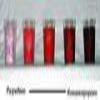### Exact Dilutions

##### Age 14 to 16 Challenge Level:

Which exact dilution ratios can you make using only 2 dilutions?### Triangle in a Triangle

##### Age 14 to 16 Challenge Level:

Can you work out the fraction of the original triangle that is covered by the inner triangle?### Points in Pairs

##### Age 14 to 16 Challenge Level:

In the diagram the radius length is 10 units, OP is 8 units and OQ is 6 units. If the distance PQ is 5 units what is the distance P'Q' ?### Trapezium Four

##### Age 14 to 16 Challenge Level:

The diagonals of a trapezium divide it into four parts. Can you create a trapezium where three of those parts are equal in area?### Bus Stop

##### Age 14 to 16 Challenge Level:

Two buses leave at the same time from two towns Shipton and Veston on the same long road, travelling towards each other. At each mile along the road are milestones. The buses' speeds are constant. . . .### Slippage

##### Age 14 to 16 Challenge Level:

A ladder 3m long rests against a wall with one end a short distance from its base. Between the wall and the base of a ladder is a garden storage box 1m tall and 1m high. What is the maximum distance. . . .### Another Triangle in a Triangle

##### Age 16 to 18 Challenge Level:

Can you work out the fraction of the original triangle that is covered by the green triangle?### Sitting Pretty

##### Age 14 to 16 Challenge Level:

A circle of radius r touches two sides of a right angled triangle, sides x and y, and has its centre on the hypotenuse. Can you prove the formula linking x, y and r?### From All Corners

##### Age 14 to 16 Challenge Level:

Straight lines are drawn from each corner of a square to the mid points of the opposite sides. Express the area of the octagon that is formed at the centre as a fraction of the area of the square.### Golden Thoughts

##### Age 14 to 16 Challenge Level:

Rectangle PQRS has X and Y on the edges. Triangles PQY, YRX and XSP have equal areas. Prove X and Y divide the sides of PQRS in the golden ratio.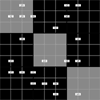### Ratio Sudoku 1

##### Age 11 to 16 Challenge Level:

A Sudoku with clues as ratios.### Rhombus in Rectangle

##### Age 14 to 16 Challenge Level:

Take any rectangle ABCD such that AB > BC. The point P is on AB and Q is on CD. Show that there is exactly one position of P and Q such that APCQ is a rhombus.### Circuit Training

##### Age 14 to 16 Challenge Level:

Mike and Monisha meet at the race track, which is 400m round. Just to make a point, Mike runs anticlockwise whilst Monisha runs clockwise. Where will they meet on their way around and will they ever. . . .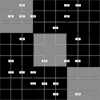### Ratio Sudoku 2

##### Age 11 to 16 Challenge Level:

A Sudoku with clues as ratios.### Star Gazing

##### Age 14 to 16 Challenge Level:

Find the ratio of the outer shaded area to the inner area for a six pointed star and an eight pointed star.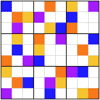### Ratio Sudoku 3

##### Age 11 to 16 Challenge Level:

A Sudoku with clues as ratios or fractions.### Orbiting Billiard Balls

##### Age 14 to 16 Challenge Level:

What angle is needed for a ball to do a circuit of the billiard table and then pass through its original position?### A Scale for the Solar System

##### Age 14 to 16 Challenge Level:

The Earth is further from the Sun than Venus, but how much further? Twice as far? Ten times?### Semi-square

##### Age 14 to 16 Challenge Level:

What is the ratio of the area of a square inscribed in a semicircle to the area of the square inscribed in the entire circle?### Speeding Boats

##### Age 14 to 16 Challenge Level:

Two boats travel up and down a lake. Can you picture where they will cross if you know how fast each boat is travelling?### Around and Back

##### Age 14 to 16 Challenge Level:

A cyclist and a runner start off simultaneously around a race track each going at a constant speed. The cyclist goes all the way around and then catches up with the runner. He then instantly turns. . . .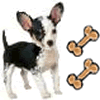### Ratio Pairs 3

##### Age 11 to 16 Challenge Level:

Match pairs of cards so that they have equivalent ratios.